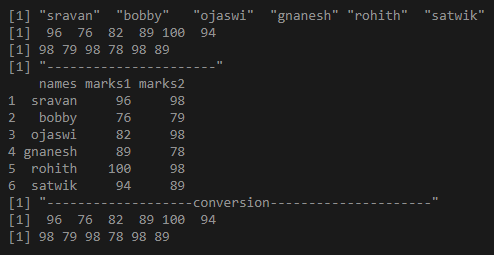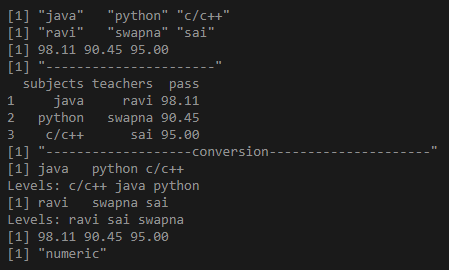Open In App

# Convert DataFrame to vector in R

In this article, we will discuss how a dataframe can be converted to a vector in R. For the Conversion of dataframe into a vector, we can simply pass the dataframe column name as [[index]].

Approach:

We are taking a column in the dataframe and passing it into another variable by the selection method. Selection method can be defined as choosing a column from a data frame using ” [[]]”.

• Create a dataframe
• Apply selection process on the columns in a dataframe which is created.
• Verify the resultant variable.

Syntax:

convert_data = dataframe_name[[‘column_name’]]

Given below are implementations using this approach to produce this functionality.

Example 1:

## R

 `# creating a vector for names``names=``c``(``'sravan'``,``'bobby'``,``'ojaswi'``,``'gnanesh'``,``'rohith'``,``'satwik'``)`` ` `# creating vector for marks 1``marks1=``c``(96,76,82,89,100,94)`` ` `# creating vector for marks 2``marks2=``c``(98,79,98,78,98,89)`` ` `# display all details``print``(names)``print``(marks1)``print``(marks2)``print``(``"----------------------"``)`` ` `# passing vectors to the data frame``Final_data = ``data.frame``(names,marks1,marks2)`` ` `# display data frame``print``(Final_data)``print``(``"-------------------conversion---------------------"``)`` ` `# converting marks1 dataframe column into vector``# by passing as index``convert_data1 = Final_data[[``'marks1'``]]``print``(convert_data1)`` ` `# converting marks2 dataframe column into vector ``# by passing as index``convert_data2 = Final_data[[``'marks2'``]]``print``(convert_data2)`

Output:Example 2:

## R

 `# creating a vector for subjects``subjects=``c``(``'java'``,``'python'``,``'c/c++'``)`` ` `# creating vector for teachers``teachers=``c``(``'ravi'``,``'swapna'``,``'sai'``)`` ` `# creating vector for pass percentage``pass=``c``(98.11,90.45,95)`` ` `# display all details``print``(subjects)``print``(teachers)``print``(pass)``print``(``"----------------------"``)`` ` `# passing vectors to the data frame``Final_data = ``data.frame``(subjects,teachers,pass)`` ` `# display data frame``print``(Final_data)``print``(``"-------------------conversion---------------------"``)`` ` `# converting subjects dataframe column into ``# vector by passing as index``convert_data1 = Final_data[[``'subjects'``]]``print``(convert_data1)`` ` `# converting teachers dataframe column into ``# vector by passing as index``convert_data2 = Final_data[[``'teachers'``]]``print``(convert_data2)`` ` `# converting pass dataframe column into vector``# by passing as index``convert_data2 = Final_data[[``'pass'``]]``print``(convert_data2)`

Output: